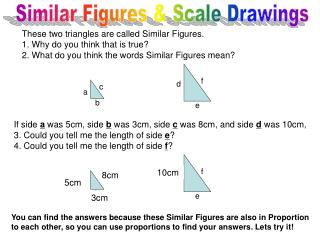DownloadDownload PresentationSimilar Figures & Scale Drawings

# Similar Figures & Scale Drawings

Télécharger la présentation## Similar Figures & Scale Drawings

- - - - - - - - - - - - - - - - - - - - - - - - - - - E N D - - - - - - - - - - - - - - - - - - - - - - - - - - -
##### Presentation Transcript

1. f f d d c c a a b b e e Similar Figures & Scale Drawings These two triangles are called Similar Figures. 1. Why do you think that is true? 2. What do you think the words Similar Figures mean? If side a was 5cm, side b was 3cm, side c was 8cm, and side d was 10cm, 3. Could you tell me the length of side e? 4. Could you tell me the length of side f? 10cm 8cm 5cm 3cm You can find the answers because these Similar Figures are also in Proportion to each other, so you can use proportions to find your answers. Lets try it!

2. In your ISN... • If the two triangles are similar, which side is congruent to • AB • BC • AC

3. Consensus Whiteboards

4. How to use Proportions to find missing sides or numbers. 10cm 8cm 5cm 3cm Divide with remaining number = Multiply across f 6cm N Corresponding Sides 5cm 10cm 3cm • 10cm ÷ 5cm = N 3cm N N Corresponding Sides 6cm 30cm ÷ 5cm = 5. What is the measure of the side f ? 6. Write out the proportion you used to find the missing measure for side f.

5. Consensus Whiteboards!

6. Homework • Page 543 • #1 - 14

7. 2 inches Bemidji Middle School 7 inches Use the scale to find the actual size of the banner. ….(Hint- set up a proportion with similar labels instead of sides) Scale Drawing is like Similar Figures except that you only see one of the similar figures. ( Ex. A State road map )-( The map gives you a scale measurement to help you determine the actual distance. 1inch : 3miles ) Scale 1 in : 3.5 ft Model of the new School Banner 7 & 8. Set up and show the proportion and give the correct length in feet? 9 & 10. Set up and show the proportion and give the correct height in feet?

8. 6-3 Homework “Scale Drawing & Similar Figures” Name:_________________ Hour: _____ Each pair of triangles is similar. Find the missing length. Round to the nearest tenth if necessary. 2 in. 1. 2. x y 10 in. 10 in. 3cm 4cm 12cm 4 in. 3. 4. 8 cm z 6.4 cm 8 cm 30˚ 30˚ 7 cm 5 cm a 7 cm

9. The scale of a map is 2 cm : 15 km. Find the actual distance for each map distance. 5. 6cm = _________km 6. 2.1 cm = _________km 8. 17.4 cm = _________km 7. 10 mm = _________km The scale drawing has a scale of 1/2 in. : 10 ft. Find the length on the drawing for each actual length. 9. 40 ft = ________________in 10. 5 ft = __________________in 12. 3 1/2 ft = _______________in 11. 35 ft = _______________in x 5 32 80 3 8 r 15 24 16 204 . c 13. = 14. = 15. = Solve Each Proportion.

10. Sample Problems The scale of a map is 2 cm : 15 km. Find the actual distance for each map distance. 10 mm ? km 3 cm ? km 2 cm 15km 2 cm 15km = = 1. 3cm = _________km 2. 10 mm = _________km x 5 15 25 3 4 r 7 24 16 204 . c 5. = 6. = 7. = 3 • 15 ÷ 2 = 22.5 22.5 km 1 cm ? km 1 • 15 ÷ 2 = 7.5 7.5 km The scale drawing has a scale of 1/2 in. : 10 ft. Find the length on the drawing for each actual length. 4 ? in 80 ft 2 in 0.5 in 10 ft 0.5 • 80 ÷ 10 = 4 = 3. 80ft = ________________in 2.5 2.5 in 0.5 • 70 ÷ 10 = 2.5 ? in 70 ft 0.5 in 10 ft 4. 70 ft = _______________in = Solve Each Proportion. **(Simplyify fraction and then solve.) 5 • 15 ÷ 25 = x 3 • 7 ÷ 4 = r 204 • 16 ÷ 24 = x